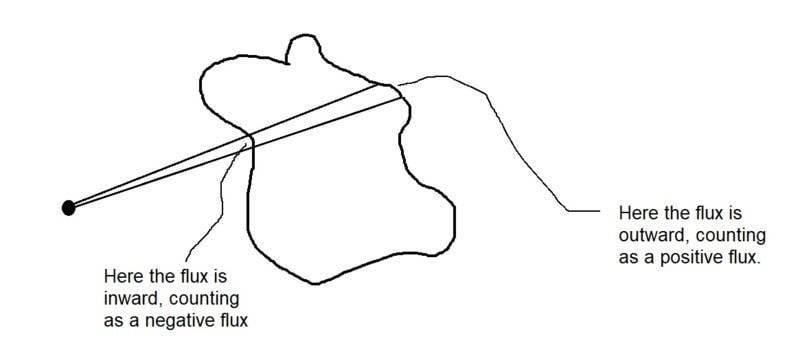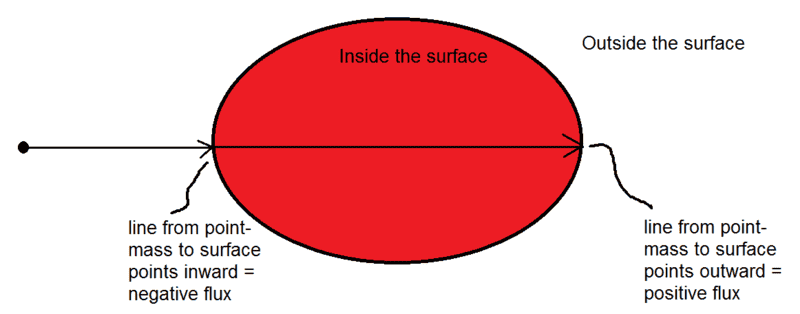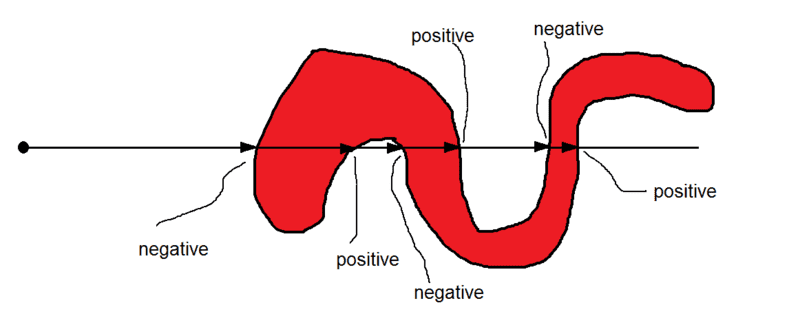# Gauss' law for gravity

• I
• TimeRip496
In summary: So the flux through this surface would be positive 3 times and negative 3 times. The total flux would be zero.To summarize, Gauss's theorem states that if a point mass is placed outside a closed surface, the total flux through the surface will be zero. If the point mass is placed inside the surface, the total flux will be negative. The cone described in the conversation is used to determine the number of times the surface is pierced by the line from the point mass, which in turn determines the direction of the flux. This is why the cone will cut the surface an odd number of times when the point mass is inside the surface and an even number of times when it is outside.In summary, Gauss's theorem uses a cone to

#### TimeRip496

"Gauss's theorem can be established as follows. consider an attracting particle of mass m at the point P, and let a cone of small solid angle ω be generated by radii through P. This cone cuts the surface at the points Q1, Q2, ... taken in order from P; the parts of the surface cut off by the cone at these points are S1, S2, ... and the outward-drawn normals are denoted n1, n2, ...

Now if P lies outside Σ (region of masses, each at points Q1, Q2, ...), the cone will cut S an even number of times, and the signs will be plus and minus alternately, so that the total normal force across S1, S2, ... will be zero. On the other hand, if P lies inside Σ, the cone will cut S an odd number of times, the sign being minus and plus alternately, so that the total normal forces across S1, S2, ... will be -mω."

Source: Spherical harmonic T.M.Macrobert

Help with this text! I don't understand the second paragraph as to why the cone will cut S an odd number of times, the sign being minus and plus alternately when P lies inside Σ vs when P lies outside Σ. I know the cone is just a mathematical object but I can't follow the author thought process here. How did he use the cone to cut the surface at Q1, Q2, ... ? Why will the normal force across S due to particle at P be zero when P is outside the mass but not inside the mass?Above is a picture illustrating the point. You have a point mass on the left, and a surface drawn on the right. The flux through a section of a closed surface is positive if the vector from the mass to the section of the surface points outward. The flux is negative if it points inward.

If the point mass is outside the surface, the positive flux through one section is canceled by the negative flux through another section. So the total flux adds up to zero. If the point mass is inside the surface, then you just have outward flux (positive).

You can make the surface more complicated by putting in "folds" in the surface. But each fold will have cancellation of the positive and negative fluxes, so folds don't change the total flux.

If the point mass is inside the surface, you have, for each cone coming away from the mass, one section with outward flux, plus 2 sections (one outward and one inward) for each fold. So the total number of sections intersecting the cone is odd.

If the point mass is outside the surface, you have two sections (one with positive flux and one with negative flux) plus 2 more for each fold. So the total number is even.

#### Attachments

stevendaryl said:
View attachment 231296

Above is a picture illustrating the point. You have a point mass on the left, and a surface drawn on the right. The flux through a section of a closed surface is positive if the vector from the mass to the section of the surface points outward. The flux is negative if it points inward.

If the point mass is outside the surface, the positive flux through one section is canceled by the negative flux through another section. So the total flux adds up to zero. If the point mass is inside the surface, then you just have outward flux (positive).

You can make the surface more complicated by putting in "folds" in the surface. But each fold will have cancellation of the positive and negative fluxes, so folds don't change the total flux.

If the point mass is inside the surface, you have, for each cone coming away from the mass, one section with outward flux, plus 2 sections (one outward and one inward) for each fold. So the total number of sections intersecting the cone is odd.

If the point mass is outside the surface, you have two sections (one with positive flux and one with negative flux) plus 2 more for each fold. So the total number is even.
I am sorry I don't quite get the direction of the flux part. For the "flux through a section of a closed surface is positive if the vector from the mass to the section of the surface points outward. The flux is negative if it points inward.", how are the direction for the flux determined? How do I know whether the vector is pointing outwards vs inwards? If we are looking at gravity, should't the direction of flux be just one as gravity only attracts and flux is just the amount of field lines passing through that surface?

TimeRip496 said:
I am sorry I don't quite get the direction of the flux part. For the "flux through a section of a closed surface is positive if the vector from the mass to the section of the surface points outward. The flux is negative if it points inward.", how are the direction for the flux determined? How do I know whether the vector is pointing outwards vs inwards? If we are looking at gravity, should't the direction of flux be just one as gravity only attracts and flux is just the amount of field lines passing through that surface?

A closed surface such as a balloon has an inside and an outside. The line from the point mass to a point on the surface has a direction. If the line is pointing inward, toward the inside of the surface, then the flux through that point on the surface is negative. If it is pointing outward, toward the outside of the surface, then the flux is positive.

.#### Attachments

•TimeRip496
In the figure below, I show an oddly-shaped surface. The line from the mass to the surface pierces the surface 6 times. 3 times the direction is inward--from outside the surface toward inside the surface. 3 times the direction is outward--from inside toward outside.#### Attachments

•TimeRip496

## 1. What is Gauss' law for gravity?

Gauss' law for gravity is a fundamental law in physics that relates the distribution of mass to the gravitational field it creates. It states that the flux of the gravitational field through a closed surface is proportional to the total mass enclosed by that surface.

## 2. How is Gauss' law for gravity derived?

Gauss' law for gravity is derived from Newton's law of universal gravitation and the principle of superposition. By considering a small volume element within a larger mass distribution, the gravitational field at a point can be found by integrating the contributions of all the nearby mass elements.

## 3. What is the significance of Gauss' law for gravity?

Gauss' law for gravity is important because it provides a mathematical relationship between the distribution of mass and the gravitational field it creates. This allows for the calculation of gravitational fields in complex systems, such as planetary orbits or the motion of galaxies.

## 4. Is Gauss' law for gravity applicable in all situations?

No, Gauss' law for gravity is only applicable in situations where the mass distribution is spherically symmetric. In other situations, such as non-uniform mass distributions or systems with multiple masses, other laws and equations must be used to calculate the gravitational field.

## 5. Can Gauss' law for gravity be used to calculate the strength of the gravitational force between two objects?

Yes, Gauss' law for gravity can be used to calculate the strength of the gravitational force between two objects by considering the flux of the gravitational field through a sphere centered on the objects. However, other equations, such as Newton's law of universal gravitation or the formula for gravitational potential energy, may be more practical for this purpose.